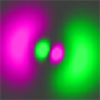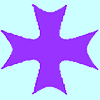# Resources tagged with: Polar coordinates

There are 5 NRICH Mathematical resources connected to Polar coordinates, you may find related items under Coordinates, Functions and Graphs.

Broad Topics > Coordinates, Functions and Graphs > Polar coordinates### Polar Bearings

##### Age 16 to 18Challenge Level

What on earth are polar coordinates, and why would you want to use them?### Bent Out of Shape

##### Age 14 to 18Challenge Level

An introduction to bond angle geometry.### An Introduction to Polar Coordinates

##### Age 14 to 18

This introduction to polar coordinates describes what is an effective way to specify position. This article explains how to convert between polar and cartesian coordinates and also encourages the. . . .### Polar Flower

##### Age 16 to 18Challenge Level

This polar equation is a quadratic. Plot the graph given by each factor to draw the flower.### Maltese Cross

##### Age 16 to 18Challenge Level

Sketch the graph of $xy(x^2 - y^2) = x^2 + y^2$ consisting of four curves and a single point at the origin. Convert to polar form. Describe the symmetries of the graph.